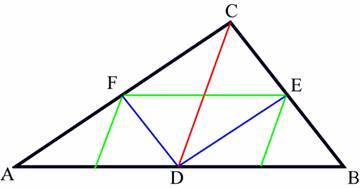favorite We need a little bit of your help to keep things running, click on this banner to learn more
Problems

# Triangle PartitioningA triangle can be divided into two triangles by drawing a median on its largest edge (In the figure above such a division is shown with the red line). Then the smaller two triangles can be divided in similar fashion into four triangles (Shown in the picture with blue lines). This process can continue forever.

Some mathematicians have found that we have only some "styles" of triangles they only differs in sizes when we split triangle into small ones in the method specified above. So now given the length of the sides of the triangle your job is to find out how many different styles of small triangles do we have. Two triangles are of same style when they are similar triangles.

Input

First line of the input file contains an integer n (0 < n < 35) that indicates how many lines of inputs are there. Each line contains three integers a, b, c (0 < a, b, c < 100) which indicates the sides of a valid triangle. A valid triangle means a real triangle with positive area.

Output

For each line of input you should produce one line of output, which contains the serial of output followed by an integer t, which indicates the number of different styles of small triangles, formed for that particular triangle. Look at the output for sample input for details. You can safely assume that for any triangle t will be less than 100.

Time limit 1 second
Memory limit 128 MiB
Input example #1
```2
3 4 5
12 84 90
```
Output example #1
```Triangle 1: 3
Triangle 2: 41
```